# 【深度学习基础】第四十六课：Beam Search

## Seq2Seq，Beam Search

Posted by x-jeff on February 23, 2021

【深度学习基础】系列博客为学习Coursera上吴恩达深度学习课程所做的课程笔记。

# 1.Seq2Seq模型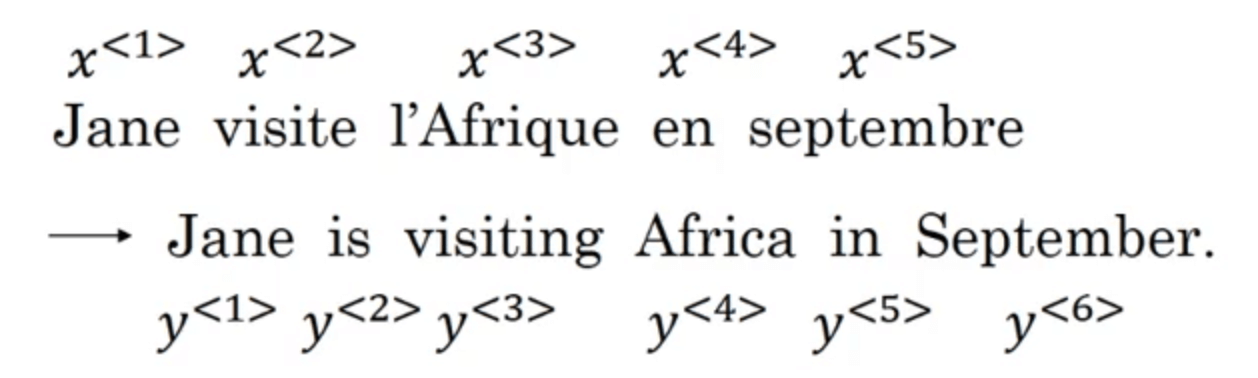1. Sutskever I, Vinyals O, Le Q V. Sequence to sequence learning with neural networks[J]. arXiv preprint arXiv:1409.3215, 2014.

2. Cho K, Van Merriënboer B, Gulcehre C, et al. Learning phrase representations using RNN encoder-decoder for statistical machine translation[J]. arXiv preprint arXiv:1406.1078, 2014.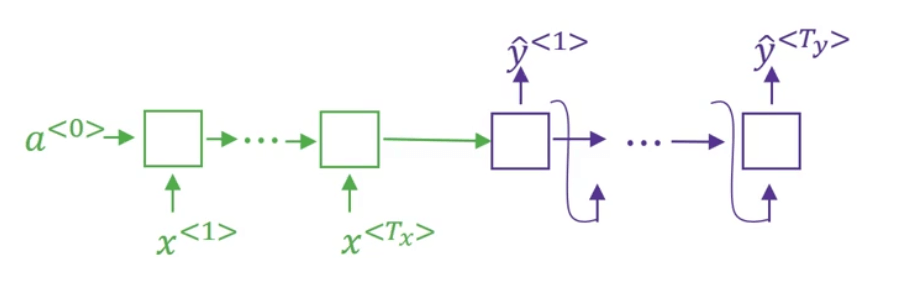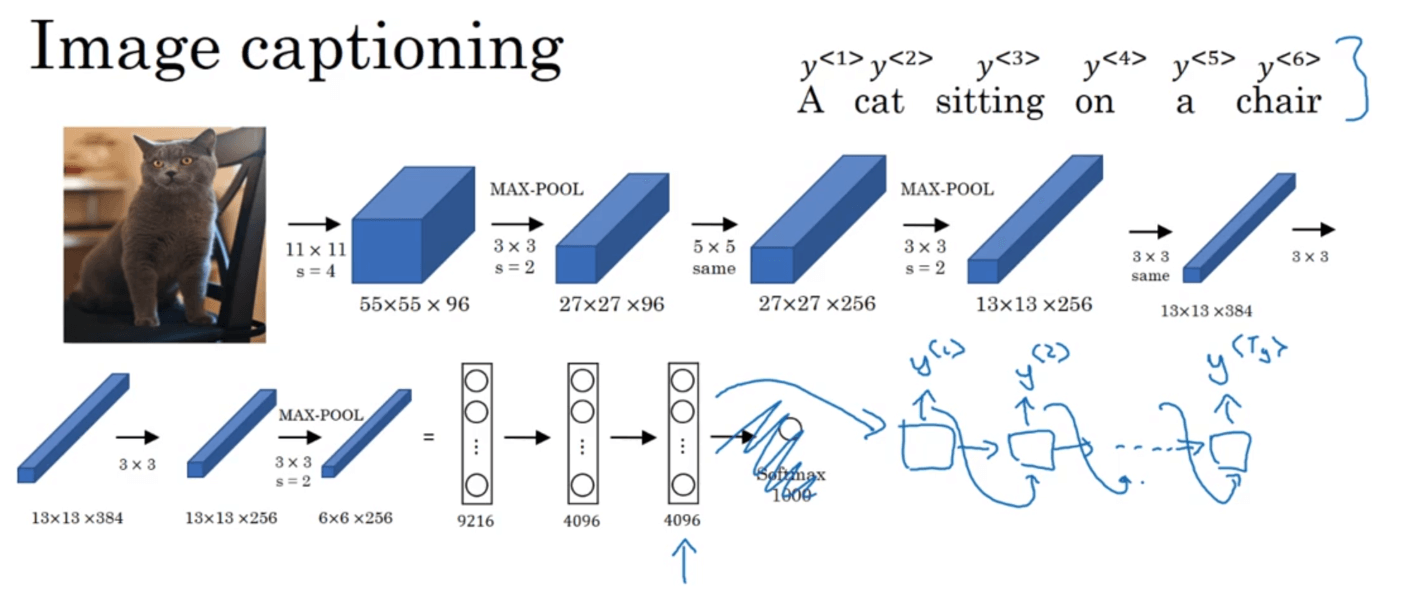image captioning相关文献：

• Mao J, Xu W, Yang Y, et al. Deep captioning with multimodal recurrent neural networks (m-rnn)[J]. arXiv preprint arXiv:1412.6632, 2014.

• Vinyals O, Toshev A, Bengio S, et al. Show and tell: A neural image caption generator[C]//Proceedings of the IEEE conference on computer vision and pattern recognition. 2015: 3156-3164.

• Karpathy A, Fei-Fei L. Deep visual-semantic alignments for generating image descriptions[C]//Proceedings of the IEEE conference on computer vision and pattern recognition. 2015: 3128-3137.

# 2.Beam Search

$\arg \max \limits_{y^{<1>},...,y^{<T_y>}}P(y^{<1>},...,y^{<T_y>} \mid x)$

Beam Search就是可以帮助我们实现这一目标的最常用的方法之一。Beam Search实现步骤见下：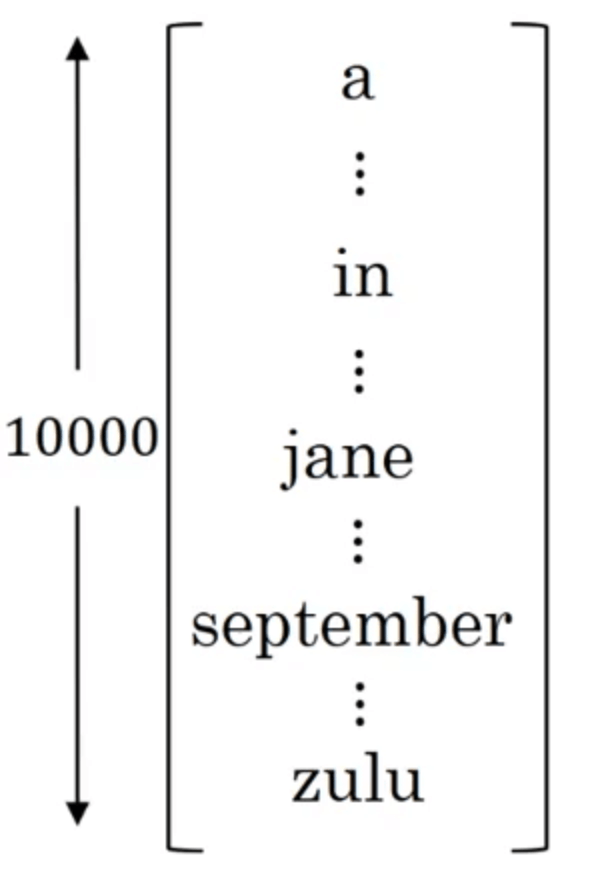Beam Search的第一步我们使用如下的网络部分（绿色是编码部分，紫色是解码部分）来评估第一个单词的概率（即$P(y^{<1>} \mid x)$，$x$为输入的法语句子）：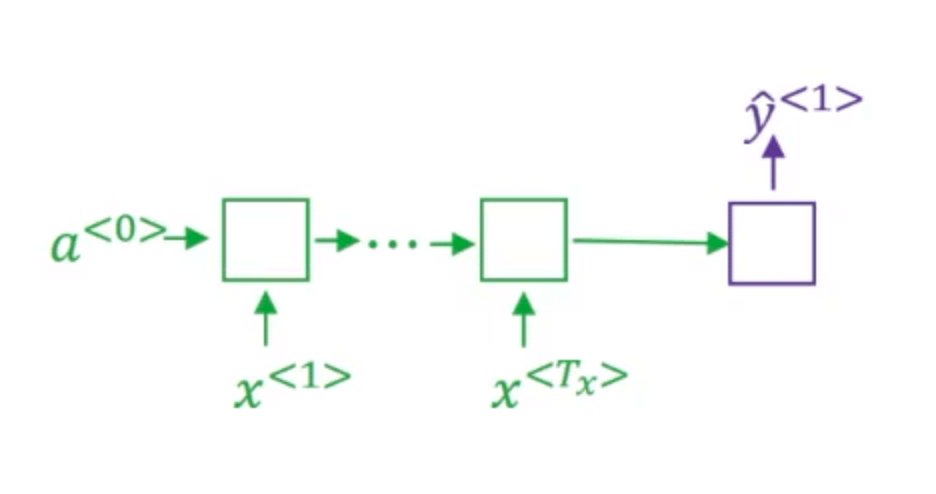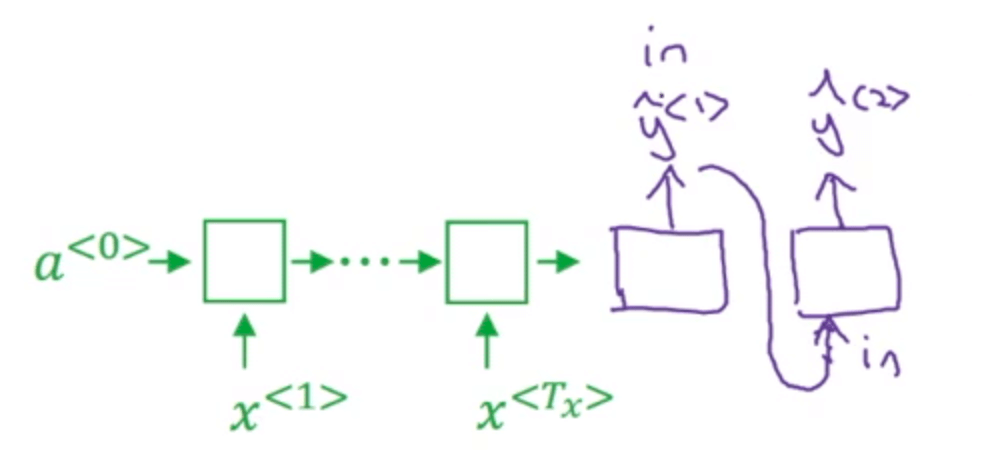$P(y^{<1>},y^{<2>} \mid x)=P(y^{<1>} \mid x) P(y^{<2>} \mid x,y^{<1>})$

1. 第二个单词=september $\mid$ 第一个单词=in
2. 第二个单词=is $\mid$ 第一个单词=jane
3. 第二个单词=visits $\mid$ 第一个单词=jane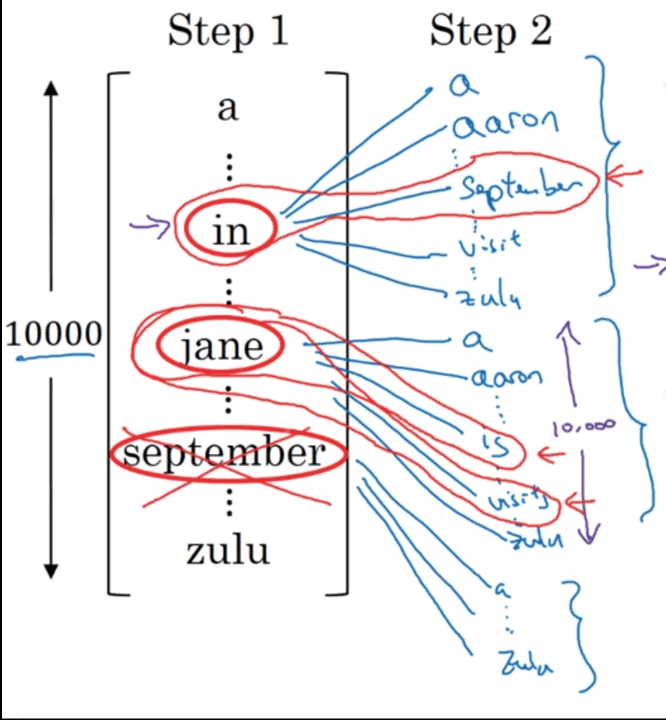Beam Search的第三步和第二步类似：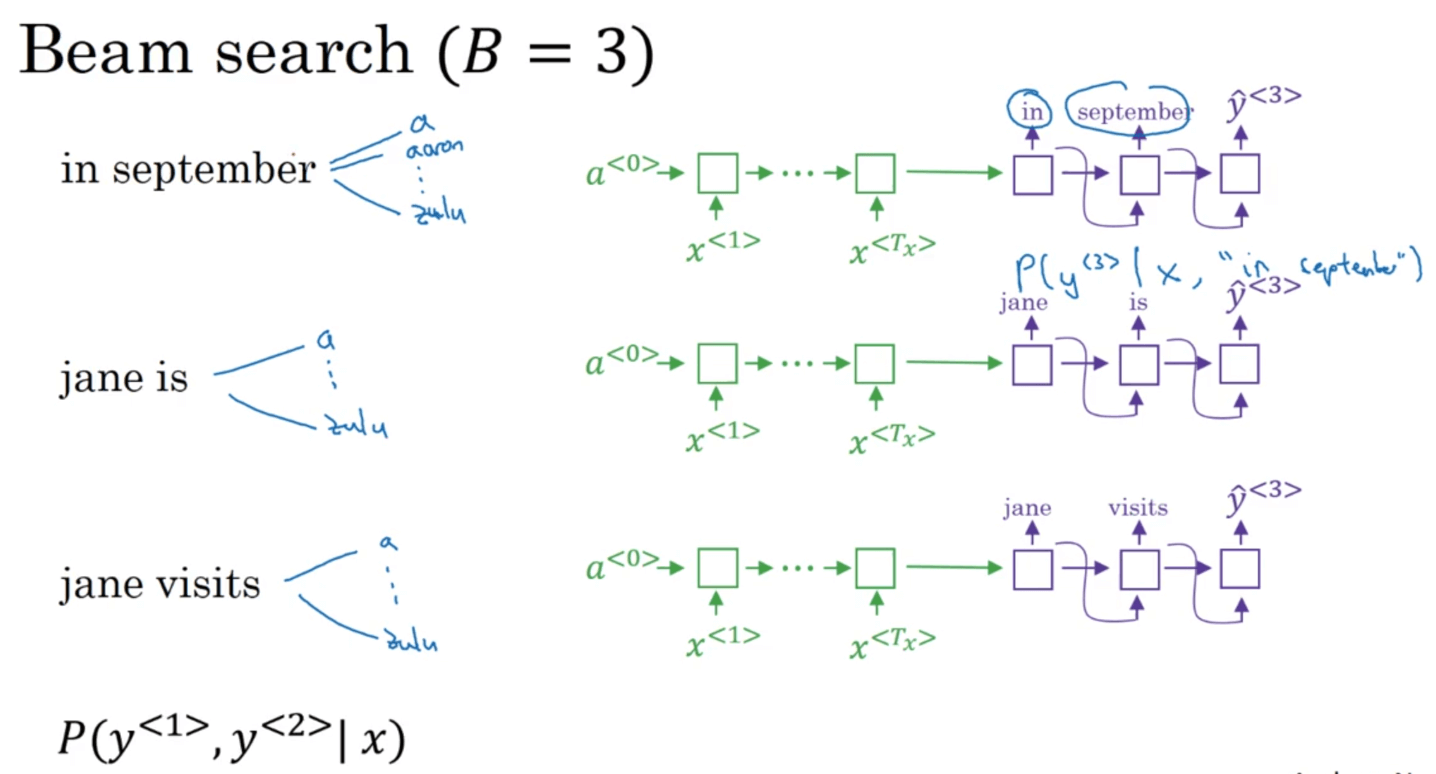# 3.Beam Search的改进

## 3.1.Length Normalization

Beam Search的目标就是最大化下述概率：

$\arg \max \limits_{y} \prod _{t=1} ^{T_y} P(y^{<t>} \mid x,y^{<1>},...,y^{<t-1>})$

$\arg \max \limits_{y} \sum _{t=1} ^{T_y} \log P(y^{<t>} \mid x,y^{<1>},...,y^{<t-1>})$

$\frac{1}{T_y^{\alpha}} \sum _{t=1} ^{T_y} \log P(y^{<t>} \mid x,y^{<1>},...,y^{<t-1>})$

## 3.2.Beam Width

Beam Width越大，意味着我们考虑的选择越多，找到的句子可能就会越好，但是算法的计算代价也会越大，算法运行更慢。

# 4.误差分析

1. $P(y^* \mid x) > P(\hat y \mid x)$，说明问题出自Beam Search算法。
2. $P(y^* \mid x) \leqslant P(\hat y \mid x)$，说明问题出自RNN网络。